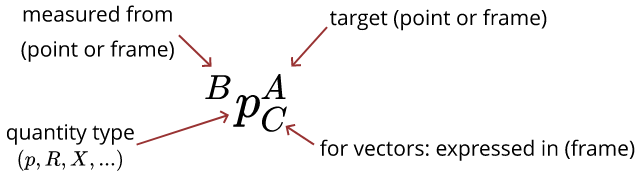Spatial Algebra

# Drake (Robot) §

Learned from the MIT Robot Manipulation course.So there are two things: points and frames.

• Points don’t have orientation?

So more precisely, we have 2 types:

1. point : You write , which means point expressed in frame
2. Rotation and Tranfsformation (rotation + translation): , , where you are going from Frame to

Note

In the past, working on the F1TENTH, I never really had to learn about points. Just learn about the transforms to go between different frames. Actually, the racing line points are points! And you transform them to go from one frame to another.

A frame  can be specified completely by a position and rotation measured from another frame. Taken together, we call the position and rotation a spatial pose, or just pose.

• A transform is the verb form of pose

In Drake we use RigidTransform to represent a pose/transform, and denote it with the letter .

Some examples:

• is the position of point  measured from point , expressed in frame   -  is the pose of frame  measured from frame . When we talk about the pose of an object , without mentioning a reference frame explicitly, we mean where  is the body frame of the object

In drake code: The Drake documentation also discusses how to use this notation in code. In short, is written p_BA_C as R_BA, as X_BA